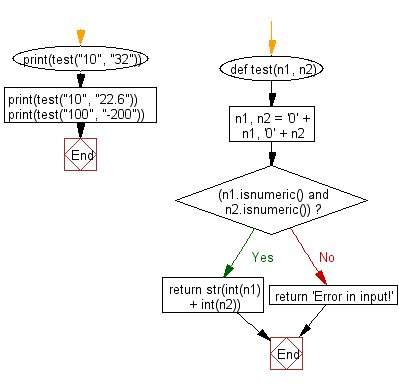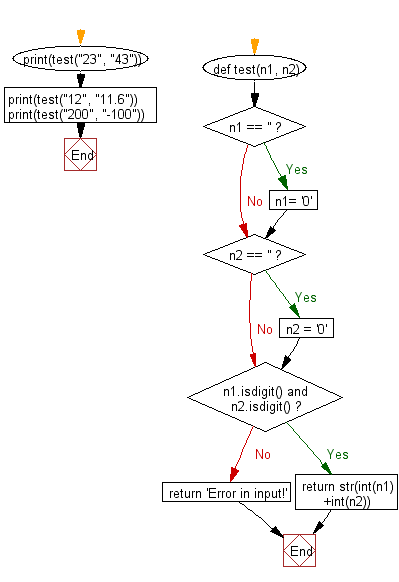﻿ Python: Add two strings as they are numbers (Positive integer values) - w3resource# Python: Add two strings as they are numbers (Positive integer values)

## Python String: Exercise-101 with Solution

Write a Python program to add two strings as they are numbers (Positive integer values). Return a message if the numbers are string.

Sample Solution-1:

Python Code:

``````def test(n1, n2):
n1, n2 = '0' + n1, '0' + n2
if (n1.isnumeric() and n2.isnumeric()):
return str(int(n1) + int(n2))
else:
return 'Error in input!'
print(test("10", "32"))
print(test("10", "22.6"))
print(test("100", "-200"))
```
```

Sample Output:

```42
Error in input!
Error in input!
```

Flowchart:## Visualize Python code execution:

The following tool visualize what the computer is doing step-by-step as it executes the said program:

Sample Solution-2:

Python Code:

``````def test(n1, n2):
if n1 == '':
n1= '0'
if n2 == '':
n2 = '0'
if n1.isdigit() and n2.isdigit():
return str(int(n1)+int(n2))
return 'Error in input!'
print(test("23", "43"))
print(test("12", "11.6"))
print(test("200", "-100"))
```
```

Sample Output:

```66
Error in input!
Error in input!
```

Flowchart:## Visualize Python code execution:

The following tool visualize what the computer is doing step-by-step as it executes the said program:

Python Code Editor:

Have another way to solve this solution? Contribute your code (and comments) through Disqus.

What is the difficulty level of this exercise?

Test your Programming skills with w3resource's quiz.

﻿

## Python: Tips of the Day

Check if a given key already exists in a dictionary:

In is the intended way to test for the existence of a key in a dict.

```d = {"key1": 10, "key2": 23}

if "key1" in d:
print("this will execute")

if "nonexistent key" in d:
print("this will not")
```

If you wanted a default, you can always use dict.get():

```d = dict()

for i in range(100):
key = i % 10
d[key] = d.get(key, 0) + 1
```

and if you wanted to always ensure a default value for any key you can either use dict.setdefault() repeatedly or defaultdict from the collections module, like so:

```from collections import defaultdict

d = defaultdict(int)

for i in range(100):
d[i % 10] += 1
```

but in general, the in keyword is the best way to do it.

Ref: https://bit.ly/2XPMRyz